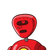# Let A be the area between co-ordinate axis, y2=x−1,x2=y−1 and the line which makes the shortest distance between two parabolas and

Let A be the area between co-ordinate axis, y2=x−1,x2=y−1 and the line which makes the shortest distance between two parabolas and A be the area between x=0,x2=y−1,x=y and the shortest distance between y2=x−1andx2=y−1,

### 1 thought on “Let A be the area between co-ordinate axis, y2=x−1,x2=y−1 and the line which makes the shortest distance between two parabolas and”

1.Notice that these are inverse functions of each other, you can swap x,y to get to the second parabola.

They are mirror images with respect to line x=y.

Required point should have this slope y′=1 for its tangent at point of tangency at ends of common normal.

Take the parabola with its symmetry axis coinciding with axis.

⇒y

2

=x−1

Differentiating w.r.t x we get,

⇒2yy′=1

⇒2y=1

⇒y=

2

1

⇒(

2

1

)

2

=x−1

⇒x=

4

5

Hence the x,y coordinates are

⇒(x,y)=(

4

5

,

2

1

)=(x

1

,y

1

) (say)

and the other point of tangency is again swapped to

⇒(x

2

,y

2

)=(

2

1

,

4

5

)

Now use distance formula between them to get the minimum distance

⇒d=

(

4

5

2

1

)

2

+(

2

1

4

5

)

2

⇒d=

(

4

3

)

2

+(

4

−3

)

2

⇒d=

4

3

2